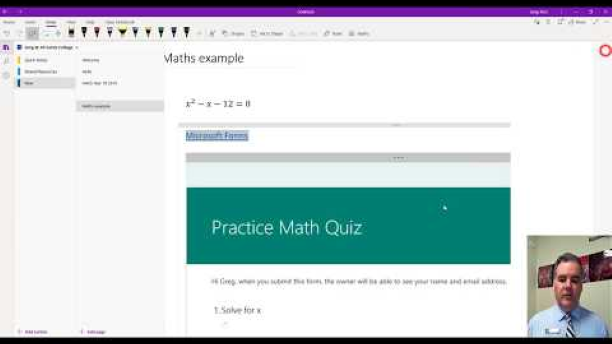# How do I generate a practice maths quiz on OneNote?

Updated
1 Views

Practice math quizzes - Students can now generate a practice math quiz directly from the Math Assistant, consisting of a set of problems like the one the student is working on. The quiz is created as a Form and embedded directly into OneNote.

Microsoft StreamMore details here

Generate a Maths quiz from OneNote